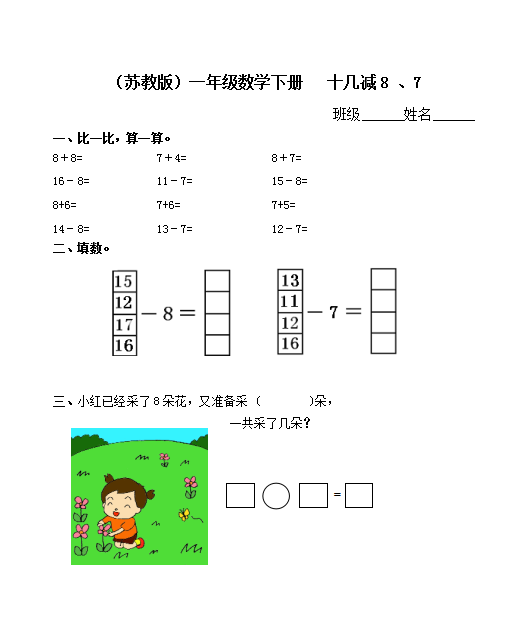（苏教版）小学一年级数学下册 十几减8 、7及答案（4页DOC文档）资料下载

（苏教版）小学一年级数学下册   十几减8 、7

8＋8=            7＋4=              8＋7=

16－8=           11－7=             15－8=

8+6=             7+6=               7+5=

14－8=           13－7=             12－7=